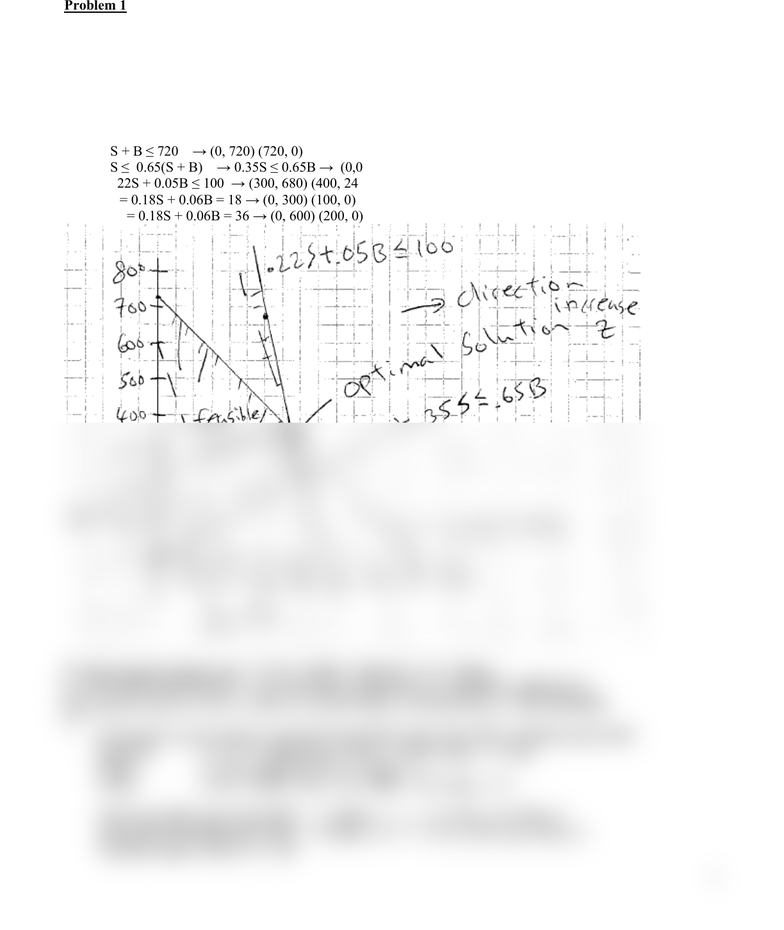Study Guides (390,000)
CA (150,000)
UTSC (10,000)
Quiz

MGOC10H3 Quiz: MGOC10H3 Quiz: assignment 2 solutionExam

Department
Management
Course Code
MGOC10H3
Professor
Vinh Quan
Study Guide
Quiz

This preview shows half of the first page. to view the full 2 pages of the document.1
Department of Management, UTSC
MGOC10 Analysis for Decision Making L01/L02 - Assignment #2 Solution
Problem 1
(a)
B = thousands of \$ invest in Bond fund, S = thousands of \$ invest in Stock fund
Max Z = 0.18S + 0.06B
S + B ≤ 720 (money available)
S ≤ 0.65(S + B) (max stock))
0.22S + 0.05B ≤ 100 (max loss)
S, B ≥ 0
(b)
S + B 720 (0, 720) (720, 0)
S 0.65(S + B) 0.35S 0.65B (0,0) (325, 175)
0.22S + 0.05B 100 (300, 680) (400, 240)
Z1 = 0.18S + 0.06B = 18 (0, 300) (100, 0)
Z2 = 0.18S + 0.06B = 36 (0, 600) (200, 0)
To obtain optimal solution solve: 0.22S + 0.05B = 100 and S + B = 720 get
S = 376.47058 or \$376,470.58 , B = 343.52941 or 343,529.41, Z = 88.37647 or \$88,376.47
Max amount can lose is 0.22S + 0.05B = 0.22(376.47058) + 0.05(34352941) = 100 or \$100,000.
(c)
From graph, current solution is optimal if slope OBJ steeper than CON1 and flatter than CON3
Objective: Z = Cs S + .06B get B = Z/.06 Cs/.06 S, slope = Cs/.06
CON1: S + B 720 get B =720 S, slope = -1
CON3: 0.22S + 0.05B = 100 B = 2000 4.4S, slope = -4.4
Want slope OBJ steeper than CON1 : Cs/0.06 -1 1 Cs/.06 get 0.06 Cs
Want slope OBJ flatter than CON3 : Cs/0.06 -4.4 4.4 Cs/.06 get 0.264 Cs
Therefore range is 0.06 Cs .264
You're Reading a Preview

Unlock to view full version